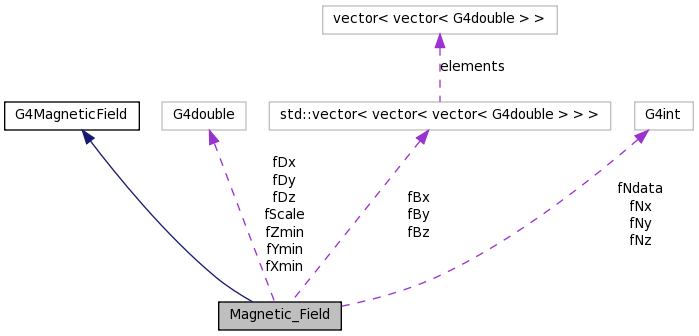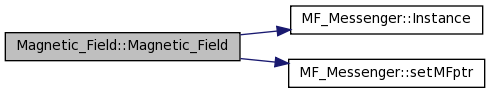# Magnetic_Field Class Reference

Declare the Magnetic_Field class. More...

`#include <Magnetic_Field.h>`

Inheritance diagram for Magnetic_Field:[legend]
Collaboration diagram for Magnetic_Field:[legend]

List of all members.

## Public Member Functions

Magnetic_Field (const char *filename, G4double scale=1.0)
Constructor for Magnetic_Field class.
void GetFieldValue (const G4double Point, G4double *Bfield) const
Member function to return the magnetic field value at a given point.
G4double getScale ()
Member function to get the magnetic field scaling factor.
G4double setScale (G4double scale=1.0)
Member function to set the magnetic field scaling factor.

## Private Attributes

vector< vector< vector
< G4double > > >
fBx
X component of field.
vector< vector< vector
< G4double > > >
fBy
Y component of field.
vector< vector< vector
< G4double > > >
fBz
Z component of field.
G4double fDx
Step size in X direction.
G4double fDy
Step size in Y direction.
G4double fDz
Step size in Z direction.
G4int fNdata
Number of grid points.
G4int fNx
Number of grid steps in X direction.
G4int fNy
Number of grid steps in Y direction.
G4int fNz
Number of grid steps in Z direction.
G4double fScale
Scale factor for calculating the field.
G4double fXmin
Starting X coordinate for grid.
G4double fYmin
Starting Y coordinate for grid.
G4double fZmin
Starting Z coordinate for grid.

## Detailed Description

Declare the Magnetic_Field class.

The Magnetic_Field class stores the grid of magnetic field values in an array of stl vectors. The routine reads a file with the magnetic field grid values and loads these into the arrays. The member function GetMagneticField is then used to return the field at a given point.

Definition at line 38 of file Magnetic_Field.h.

## Constructor & Destructor Documentation

 Magnetic_Field::Magnetic_Field ( const char * filename, G4double scale = `1.0` )

Constructor for Magnetic_Field class.

Reads the file specified by filename for the magnetic field value grid and stores this into the arrays. Also stores the scale factor scale used in scaling the magnetic field reurned by the member function.

Parameters:
 [in] filename - name of file containing the magnetic field grid [in] scale - multiplicative factor used to scale the calculated magnetic field returned by the member function GetFieldValue.

Definition at line 31 of file Magnetic_Field.cc.

References fBx, fBy, fBz, fDx, fDy, fDz, fNdata, fNx, fNy, fNz, fXmin, fYmin, fZmin, MF_Messenger::Instance(), and MF_Messenger::setMFptr().

Here is the call graph for this function:## Member Function Documentation

 void Magnetic_Field::GetFieldValue ( const G4double Point, G4double * Bfield ) const

Member function to return the magnetic field value at a given point.

Performs a simple linear interpolation over the grid of stored values which bracket the requested space point point and returns the magnetic field Bfield at that point.

Parameters:
 [in] Point - array of x, y, z, t coordinates specifying the coordinates where the field should be evaluated (time coordinate ignored in this code). [out] Bfield - pointer to a six dimensional array giving the magnetic and electric field components at Point

Definition at line 108 of file Magnetic_Field.cc.

References fBx, fBy, fBz, fDx, fDy, fDz, fNx, fNy, fNz, fScale, fXmin, fYmin, and fZmin.

 G4double Magnetic_Field::getScale ( )

Member function to get the magnetic field scaling factor.

Returns the current magnetic field scale factor.

Definition at line 194 of file Magnetic_Field.cc.

References fScale.

 G4double Magnetic_Field::setScale ( G4double scale = `1.0` )

Member function to set the magnetic field scaling factor.

The interpolated magnetic field is scaled, multiplicatively, by this factor to determine the actual field. Typically this is set to 1.0 for normal operation but can be set to any value in the range [-1.0, 1.0] for reasonable operation.

Parameters:
 [in] scale - scale factor should be in range [-1, 1]

Definition at line 188 of file Magnetic_Field.cc.

References fScale.

## Member Data Documentation

 vector< vector< vector< G4double > > > Magnetic_Field::fBx` [private]`

X component of field.

Definition at line 42 of file Magnetic_Field.h.

Referenced by GetFieldValue(), and Magnetic_Field().

 vector< vector< vector< G4double > > > Magnetic_Field::fBy` [private]`

Y component of field.

Definition at line 43 of file Magnetic_Field.h.

Referenced by GetFieldValue(), and Magnetic_Field().

 vector< vector< vector< G4double > > > Magnetic_Field::fBz` [private]`

Z component of field.

Definition at line 44 of file Magnetic_Field.h.

Referenced by GetFieldValue(), and Magnetic_Field().

 G4double Magnetic_Field::fDx` [private]`

Step size in X direction.

Definition at line 55 of file Magnetic_Field.h.

Referenced by GetFieldValue(), and Magnetic_Field().

 G4double Magnetic_Field::fDy` [private]`

Step size in Y direction.

Definition at line 56 of file Magnetic_Field.h.

Referenced by GetFieldValue(), and Magnetic_Field().

 G4double Magnetic_Field::fDz` [private]`

Step size in Z direction.

Definition at line 57 of file Magnetic_Field.h.

Referenced by GetFieldValue(), and Magnetic_Field().

 G4int Magnetic_Field::fNdata` [private]`

Number of grid points.

Definition at line 46 of file Magnetic_Field.h.

Referenced by Magnetic_Field().

 G4int Magnetic_Field::fNx` [private]`

Number of grid steps in X direction.

Definition at line 47 of file Magnetic_Field.h.

Referenced by GetFieldValue(), and Magnetic_Field().

 G4int Magnetic_Field::fNy` [private]`

Number of grid steps in Y direction.

Definition at line 48 of file Magnetic_Field.h.

Referenced by GetFieldValue(), and Magnetic_Field().

 G4int Magnetic_Field::fNz` [private]`

Number of grid steps in Z direction.

Definition at line 49 of file Magnetic_Field.h.

Referenced by GetFieldValue(), and Magnetic_Field().

 G4double Magnetic_Field::fScale` [private]`

Scale factor for calculating the field.

Definition at line 59 of file Magnetic_Field.h.

Referenced by GetFieldValue(), getScale(), and setScale().

 G4double Magnetic_Field::fXmin` [private]`

Starting X coordinate for grid.

Definition at line 51 of file Magnetic_Field.h.

Referenced by GetFieldValue(), and Magnetic_Field().

 G4double Magnetic_Field::fYmin` [private]`

Starting Y coordinate for grid.

Definition at line 52 of file Magnetic_Field.h.

Referenced by GetFieldValue(), and Magnetic_Field().

 G4double Magnetic_Field::fZmin` [private]`

Starting Z coordinate for grid.

Definition at line 53 of file Magnetic_Field.h.

Referenced by GetFieldValue(), and Magnetic_Field().

The documentation for this class was generated from the following files: